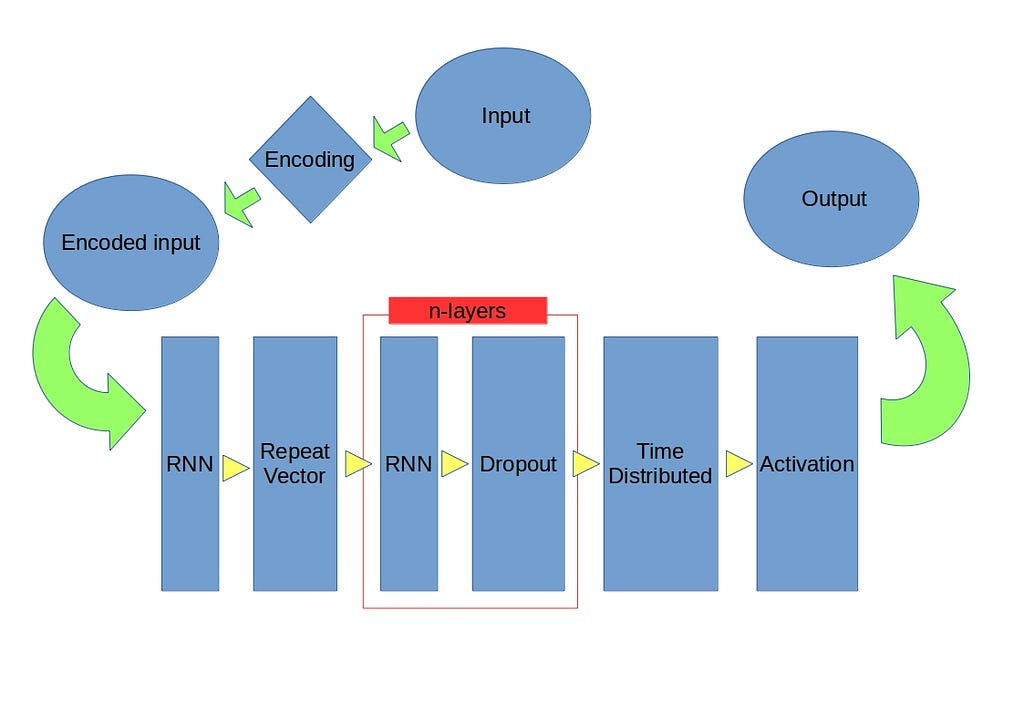# Building a calculator with seq2seq model (Keras)

A toy example, and tutorial, for using seq2seq in Keras.

Seq2seq stands for “sequence to sequence”. This kind of model is used, like that name says, to map one type of sequence to another type of sequence. For example this is used in translation where the sequences consist of texts that need to be translated from one language to another. For an in depth explanation of seq2seq [Make article].

Several examples in this domain can be found on the internet, however language is harder to train and thus seems less suited for entry level experimentation. This is the reason why I will make a model that can calculate, instead of translate. The basic principles for both calculation and translation are the same, the “only” difference being the symbols used.

#### Data generation

The input consists of the following: “number” + “sign” + “number”, which then equals “number”. The pluses stand for concatenation in this case. This is also quite literal what happens in the code, as we convert the numbers to strings, and concatenate these with the sign in the middle. So we end up with for example “55+49” as input and “104” as correct output.

The seq2seq model does not care what symbols we use, we could use “%%+\$(” with “!)\$” and the model would achieve similar results. So what we do is encode our symbols. This is done by looping over the symbols we will use, and assigning a number to each one. During the encoding step of the algorithm we create vectors of the same length as the number of symbols we have, and use a one-hot encoding for each symbol according the the assigned number. For numbers this is straight forward and we end up with something like {0:0, 1:1, 2:2, 3:3, 4:4, 5:5, 6:6, 7:7, 8:8, 9:9, 10:+, 11:-, 12:*, 13:/} as our encoding dictionary. So the one-hot encoding of 6 would be [0,0,0,0,0,0,1,0,0,0,0,0,0,0].

The input data is generated locally, another advantage over language. It is much easier to generate calculations yourself, then to do this with language. The maximum input length is “length_nr + 1 + length_nr” which is 7 in our case. We would like to also have calculations that are shorter. This is entirely possible, however a seq2seq requires [check] inputs of the same length. So when a calculation is smaller then length 7 we fill it up from the left with spaces, e.g. “____2+2”. After this padding with spaces we encode the input, like describe before, with every symbol being a one-hot vector.

#### The model

The model consists just of a number of RNN layers, with LSTM cells. After the first layer we use a RepeatVector layer. After the other RNN layers we use a Dropout layer. After the RNN layers we have a TimeDistributed layer .

#### The codeSource: Deep Learning on Medium